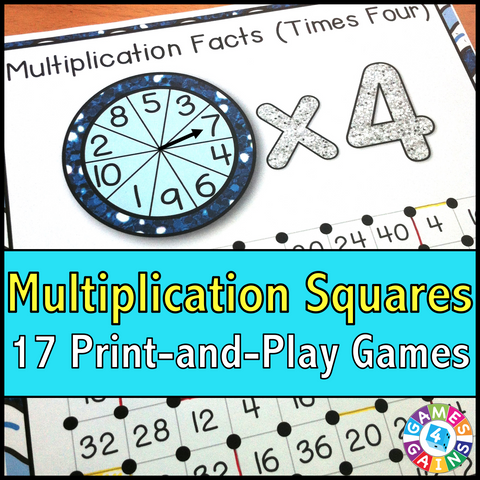## Multiplication 'Squares' Game

• \$425

These multiplication games make fact practice so much fun! This Multiplication Squares Games Pack contains 17 fun and engaging printable "squares" games to help students to practice their multiplication facts.

Each one-page multiplication game gets students practicing a different set of multiplication facts (x1, x2, x3, etc). This means that you can have each student practicing the set of facts that he/she needs the most help with!

12 Single-Fact Games
• Multiplying the numbers 1-10 by 1
• Multiplying the numbers 1-10 by 2
• Multiplying the numbers 1-10 by 3
• Multiplying the numbers 1-10 by 4
• Multiplying the numbers 1-10 by 5
• Multiplying the numbers 1-10 by 6
• Multiplying the numbers 1-10 by 7
• Multiplying the numbers 1-10 by 8
• Multiplying the numbers 1-10 by 9
• Multiplying the numbers 1-10 by 10
• Multiplying the numbers 1-10 by 11
• Multiplying the numbers 1-10 by 12

5 Mixed-Fact Games
• Multiplying the numbers 1-5 by the numbers 1-5
• Multiplying the numbers 1-5 by the numbers 6-10
• Multiplying the numbers 6-10 by the numbers 6-10
• Multiplying the numbers 1-10 by the numbers 1-10
• Multiplying by the numbers 1-12 by the numbers 1-12

These Multiplication "Squares" games are so simple to use, and take a minimal amount of prep. Simply print out the game sheet, get a paperclip and fastener, and grab a few markers, and you'll be ready to go!

To play, students will spin the spinner and solve the multiplication problem. Then, they have to find the solution on the game board and draw a line between two of the dots surrounding that number. When a student's line forms a complete square, he/she gets to capture that square. The student with the most squares at the end of the game is the winner!

Note: The spinners included on most game boards can be replaced with 10-sided and 12-sided dice. If you would prefer to use dice, I recommend these dice sets on Amazon: Mixed Dice Sets (affiliate link).

Included with this Multiplication "Squares" Game:
Instructions for play
17 Multiplication "Squares" game boards (x1, x2, x3, x4, x5, x6, x7, x8, x9, x10, x11, x12, plus five games of mixed facts)

Want to learn more? Check out the preview!